Home    |    Teacher    |    Parents    |    Glossary    |    About UsThe area of a shape is a number that tells how many square units are needed to cover the shape. Area can be measured in different units, such as square feet, square meters, or square inches.

You can find an area by drawing a shape on graph paper, and counting the squares inside the shape. But this is not very practical, so we use area formulas instead. Every polygon and circle has a formula for finding its area.

Move your mouse over each shape to see the formula for finding its area.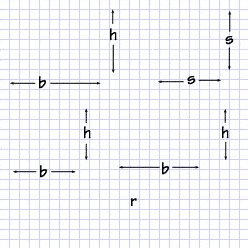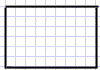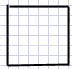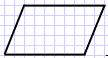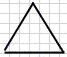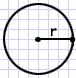Homework Help | Geometry | PolygonsEmail this page to a friendSearch·  Polygon basics·  Triangles·  Quadrilaterals·  Area of polygons     and circles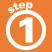First Glance In Depth Examples WorkoutArea of polygons and circles